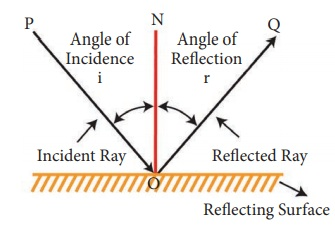Home | | Science 7th Std | Terms used in reflection of light

# Terms used in reflection of light

Incident ray: The ray of light that falls on the surface of the reflection materials. In figure, PO is the incident ray.

Terms used in reflection of light.Incident ray: The ray of light that falls on the surface of the reflection materials. In figure, PO is the incident ray.

Reflected ray: The ray of light that comes from the point when the incident ray falls on the reflection material. In the figure, OQ is the reflected ray.

Point of incidence : The point of which are incident ray strikes the reflecting surface is the point of incidence. In the figure ‘O‘ point of incidence.

Normal : The perpendicular line drawn from the point of incidence to the plane of reflecting surface is called normal. In figure, ON is the normal.

Angle of incidence: The angle formed between the incident ray PO and the normal ‘ON’ is angle of incidence. It is denoted by ∟i

Angle of reflection: The angle formed between the reflected ray OQ and the normal ON is angle of reflection. It is denoted by ∟i

Tags : Light | Term 3 Unit 1 | 7th Science , 7th Science : Term 3 Unit 1 : Light
Study Material, Lecturing Notes, Assignment, Reference, Wiki description explanation, brief detail
7th Science : Term 3 Unit 1 : Light : Terms used in reflection of light | Light | Term 3 Unit 1 | 7th Science